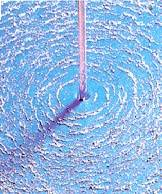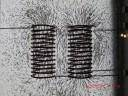# Magnetic Field using Ampere's Law

I find Ampere's Circuital Law sort of fishy. I don't understand what the actual theory proposes. And the loop that should be taken into consideration adds much to the confusion. How should we select the loop?

And in the case of a long wire we find the magnetic field around it by applying ##B.2\pi r= \mu_o i_{enc}##. So how do we find the magnetic field due to a short wire (which is not long or infinitely long)?
Using Biot Savart Law we find the magnetic field due to a short wire as ##\mu_o/4\pi r (cos\theta_1-cos\theta_2)##
where ##cos\theta_1## and ##cos\theta_2## are the angles between the length vector (towards the direction of current) and the position vector at the extreme ends.

Yes, using Biot-Savart Law is a way to go here. About integration procedures for particular examples ask in math calculus section.

jim hardy
Gold Member
Dearly Missed
I find Ampere's Circuital Law sort of fishy. I don't understand what the actual theory proposes. And the loop that should be taken into consideration adds much to the confusion. How should we select the loop?

Fishy ?

As a kid did you never tinker with iron filings and a battery?

Ampere allows one to put a number on this phenomenon...http://coe.kean.edu/~afonarev/Physics/Magnetism/Magnetic Fields and Forces-eL.htm

There's no overwhelming reason to chose any particular closed loop path in air
so i'd pick one that makes for a not-very-cumbersome integral

but in solving a practical problem like a transformer ,,https://sharepoint.umich.edu/lsa/physics/demolab/SitePages/5H15.40 - Projection of the Magnetic Field Due to a Current in a Solenoid.aspx

you'd probably find it handy to pick a path through the middle (or centroid) of its iron core.

I guess using a clamp-on ammeter sorta made it intuitive for me...

http://www.sears.com/craftsman-digital-clamp-on-ammeter/p-03482369000P
http://c.shld.net/rpx/i/s/i/spin/image/spin_prod_1113787012?hei=444&wid=444&op_sharpen=1

•I_am_learning
But I would like to know, why do we obtain the answer for a particular case (here, the magnetic field due to a long wire) using Ampere's Law. I mean if we are asked to find the magnetic field due to a short wire how do we do it? (I heard that Ampere's Law is the general rule for finding the magnetic field than the Biot-Savart Law)?

To call Ampere's law "fishy" is a very bad choice of words. Ampere's Law and Biot-Savart Law are equivivalent in magnetostatics (meaning one can be derived from another). Which one do you choose to use depends on the problem's geometry. In your example of finitely long straight wire, Biot-Savart Law is more convinient to use.

•jim hardy